# CHAPTER FIVE Liquid Extraction 1 Liquid Extraction Liquidliquid

• Slides: 28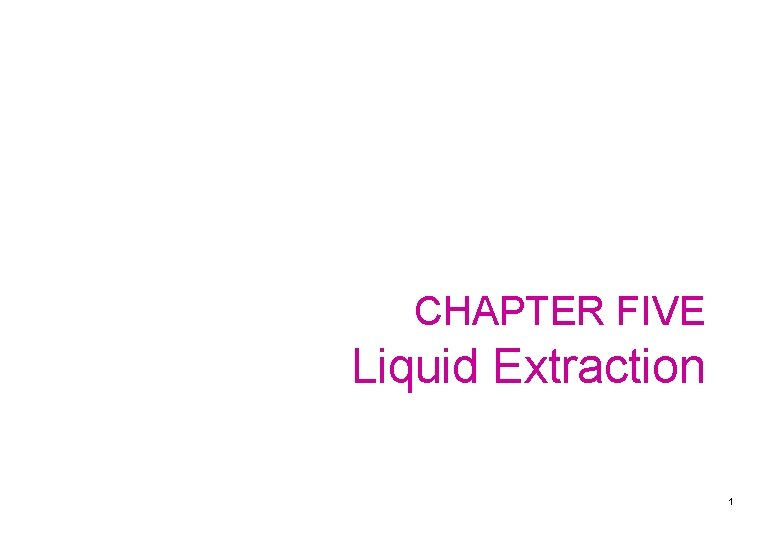CHAPTER FIVE Liquid Extraction 1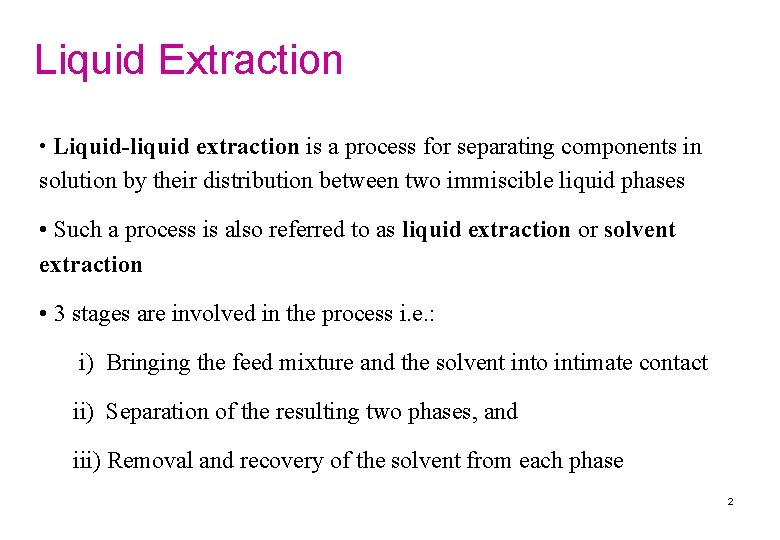Liquid Extraction • Liquid-liquid extraction is a process for separating components in solution by their distribution between two immiscible liquid phases • Such a process is also referred to as liquid extraction or solvent extraction • 3 stages are involved in the process i. e. : i) Bringing the feed mixture and the solvent into intimate contact ii) Separation of the resulting two phases, and iii) Removal and recovery of the solvent from each phase 2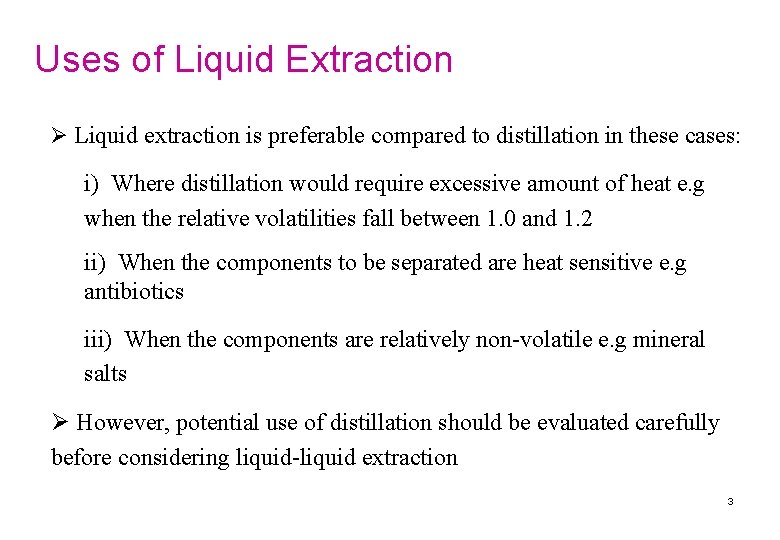Uses of Liquid Extraction Ø Liquid extraction is preferable compared to distillation in these cases: i) Where distillation would require excessive amount of heat e. g when the relative volatilities fall between 1. 0 and 1. 2 ii) When the components to be separated are heat sensitive e. g antibiotics iii) When the components are relatively non-volatile e. g mineral salts Ø However, potential use of distillation should be evaluated carefully before considering liquid-liquid extraction 3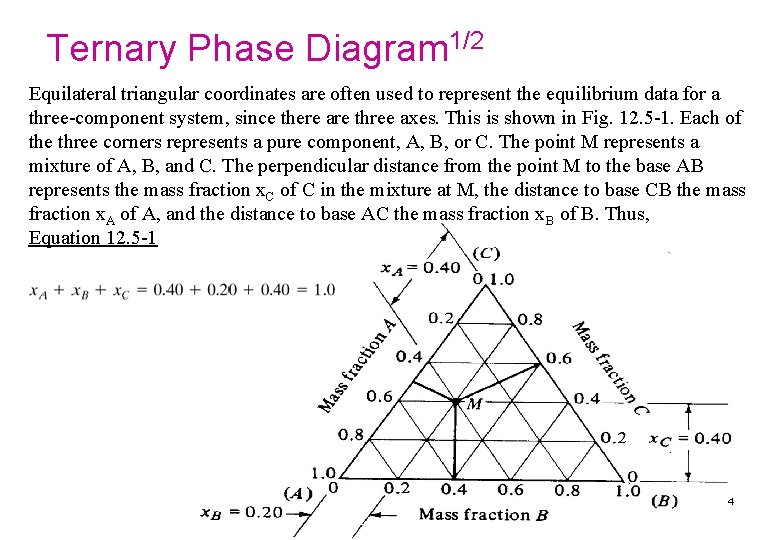Ternary Phase Diagram 1/2 Equilateral triangular coordinates are often used to represent the equilibrium data for a three-component system, since there are three axes. This is shown in Fig. 12. 5 -1. Each of the three corners represents a pure component, A, B, or C. The point M represents a mixture of A, B, and C. The perpendicular distance from the point M to the base AB represents the mass fraction x. C of C in the mixture at M, the distance to base CB the mass fraction x. A of A, and the distance to base AC the mass fraction x. B of B. Thus, Equation 12. 5 -1 4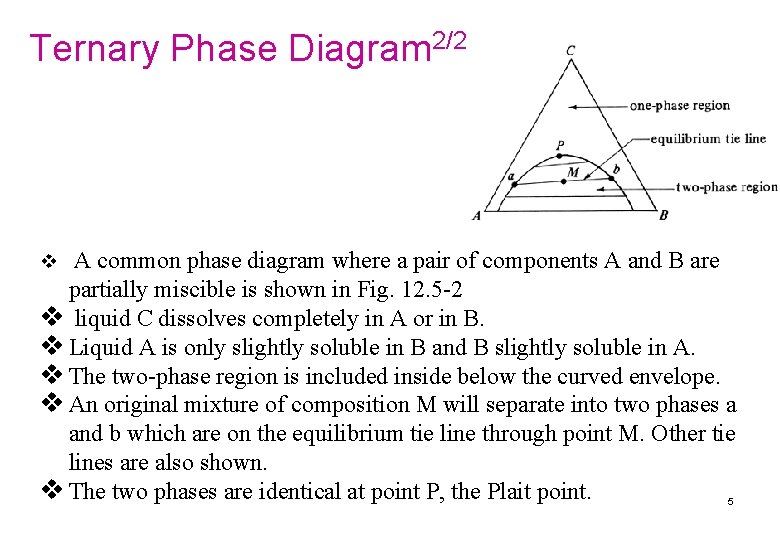Ternary Phase Diagram 2/2 v A common phase diagram where a pair of components A and B are partially miscible is shown in Fig. 12. 5 -2 v liquid C dissolves completely in A or in B. v Liquid A is only slightly soluble in B and B slightly soluble in A. v The two-phase region is included inside below the curved envelope. v An original mixture of composition M will separate into two phases a and b which are on the equilibrium tie line through point M. Other tie lines are also shown. v The two phases are identical at point P, the Plait point. 5Equilibrium data on rectangular coordinates l Since triangular diagrams have some disadvantages due to the special coordinates, a more useful method of plotting the three-component data is to use rectangular coordinates. l This is shown in Fig. 12. 5 -3 for the system acetic acid (A)– water (B)–isopropyl ether solvent (C). l The solvent pair B and C are partially miscible. l The concentration of component C is plotted on the vertical axis and that of A on the horizontal axis. The concentration of component B is obtained by difference from Eq. (12. 5 -2) or (12. 5 -3): Equation 12. 5 -2 Equation 12. 5 -3 l The two-phase region in Fig. 12. 5 -3 is inside the envelope and the one-phase region outside. l A tie line gi is shown connecting the water-rich layer i, called the raffinate layer, and the ether-rich solvent layer g, called the extract layer. l The raffinate composition is designated by x and the extract by y. Hence, the mass fraction of C is designated as y. C in the extract layer and as x. C in the raffinate layer. l To construct the tie line gi using the equilibrium y. A - x. A plot below the phase diagram, vertical lines to g and i are drawn. 6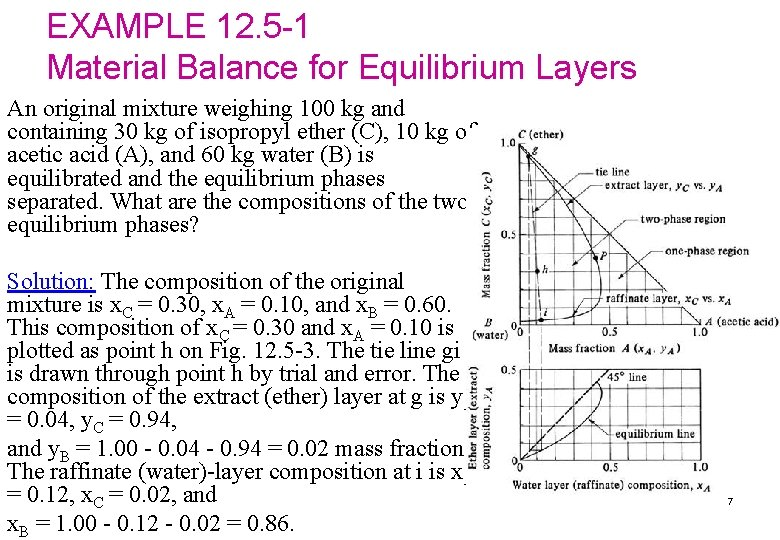EXAMPLE 12. 5 -1 Material Balance for Equilibrium Layers An original mixture weighing 100 kg and containing 30 kg of isopropyl ether (C), 10 kg of acetic acid (A), and 60 kg water (B) is equilibrated and the equilibrium phases separated. What are the compositions of the two equilibrium phases? Solution: The composition of the original mixture is x. C = 0. 30, x. A = 0. 10, and x. B = 0. 60. This composition of x. C = 0. 30 and x. A = 0. 10 is plotted as point h on Fig. 12. 5 -3. The tie line gi is drawn through point h by trial and error. The composition of the extract (ether) layer at g is y. A = 0. 04, y. C = 0. 94, and y. B = 1. 00 - 0. 04 - 0. 94 = 0. 02 mass fraction. The raffinate (water)-layer composition at i is x. A = 0. 12, x. C = 0. 02, and x. B = 1. 00 - 0. 12 - 0. 02 = 0. 86. 7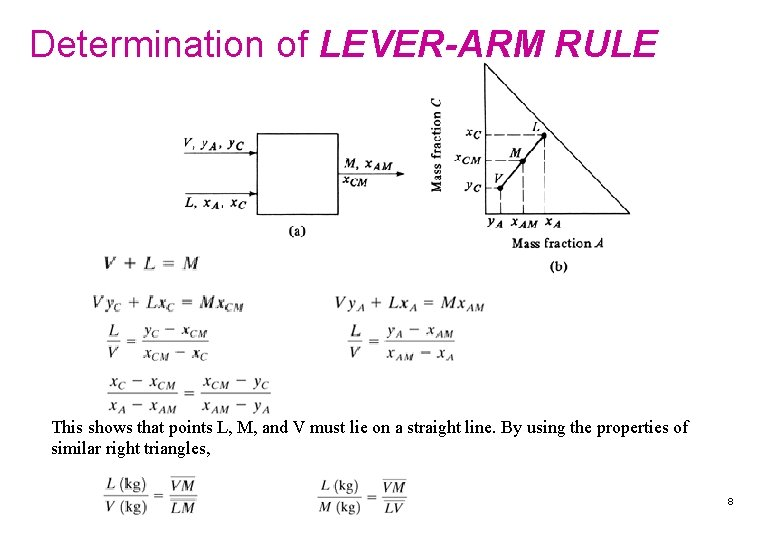Determination of LEVER-ARM RULE This shows that points L, M, and V must lie on a straight line. By using the properties of similar right triangles, 8EXAMPLE 12. 5 -2. Amounts of Phases in Solvent Extraction The compositions of the two equilibrium layers in Example 12. 5 -1 are for the extract layer (V), y. A = 0. 04, y. B = 0. 02, and y. C = 0. 94, and for the raffinate layer (L), x. A = 0. 12, x. B = 0. 86, and x. C = 0. 02. The original mixture contained 100 kg and x. AM = 0. 10. Determine the amounts of V and L. Solution: Substituting into Eq. (12. 5 -4), V + L = 100 Substituting into Eq. (12. 5 -5), where M = 100 kg and x. AM = 0. 10, V (0. 04) + L (0. 12) = 100 (0. 1) Solving the two equations simultaneously, L = 75. 0 and V = 25. 0. Alternatively, using the lever-arm rule, the distance hg in Fig. 12. 5 -3 is measured as 4. 2 units and gi as 5. 8 units. Then, by Eq. (12. 5 -11), Solving, L = 72. 5 kg and V = 27. 5 kg, which is in reasonably close agreement with the material-balance method. 9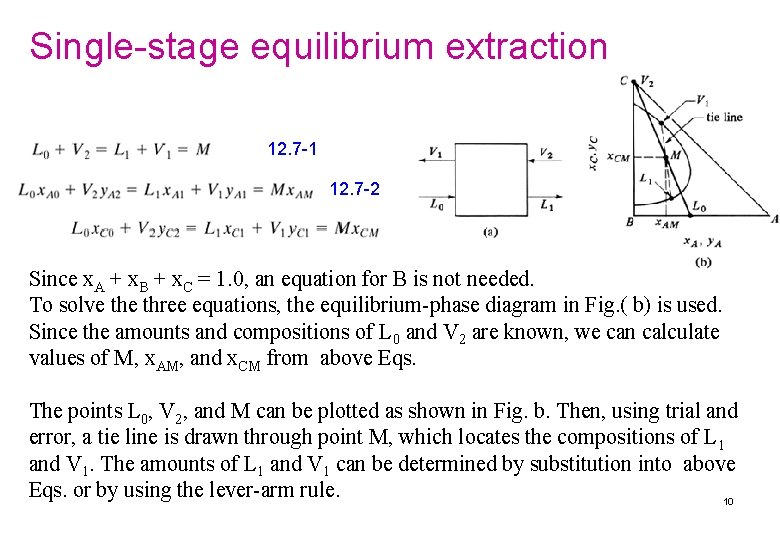Single-stage equilibrium extraction 12. 7 -1 12. 7 -2 Since x. A + x. B + x. C = 1. 0, an equation for B is not needed. To solve three equations, the equilibrium-phase diagram in Fig. ( b) is used. Since the amounts and compositions of L 0 and V 2 are known, we can calculate values of M, x. AM, and x. CM from above Eqs. The points L 0, V 2, and M can be plotted as shown in Fig. b. Then, using trial and error, a tie line is drawn through point M, which locates the compositions of L 1 and V 1. The amounts of L 1 and V 1 can be determined by substitution into above Eqs. or by using the lever-arm rule. 10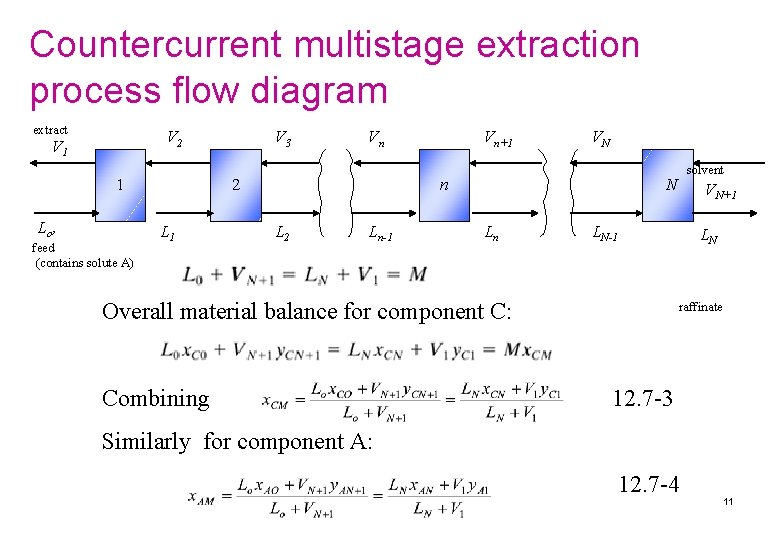Countercurrent multistage extraction process flow diagram extract V 2 V 1 1 Lo , feed (contains solute A) V 3 Vn 2 L 1 Vn+1 VN n L 2 Ln-1 N Ln VN+1 LN-1 Overall material balance for component C: Combining solvent LN raffinate 12. 7 -3 Similarly for component A: 12. 7 -4 11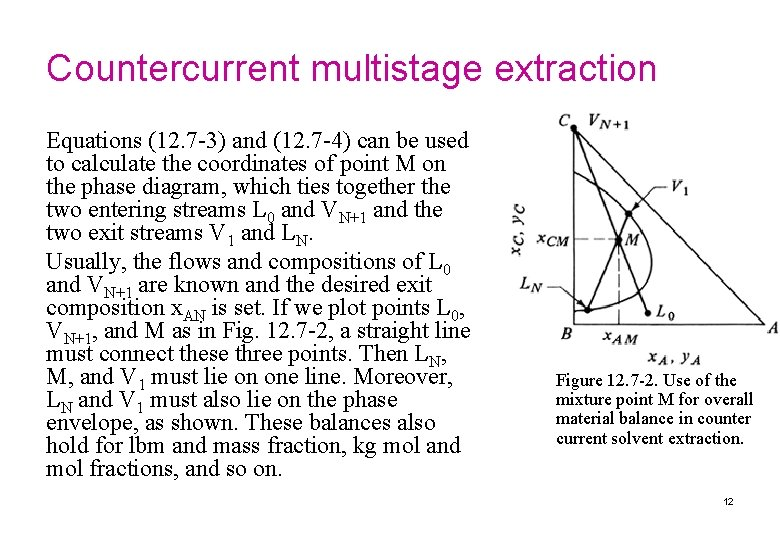Countercurrent multistage extraction Equations (12. 7 -3) and (12. 7 -4) can be used to calculate the coordinates of point M on the phase diagram, which ties together the two entering streams L 0 and VN+1 and the two exit streams V 1 and LN. Usually, the flows and compositions of L 0 and VN+1 are known and the desired exit composition x. AN is set. If we plot points L 0, VN+1, and M as in Fig. 12. 7 -2, a straight line must connect these three points. Then LN, M, and V 1 must lie on one line. Moreover, LN and V 1 must also lie on the phase envelope, as shown. These balances also hold for lbm and mass fraction, kg mol and mol fractions, and so on. Figure 12. 7 -2. Use of the mixture point M for overall material balance in counter current solvent extraction. 12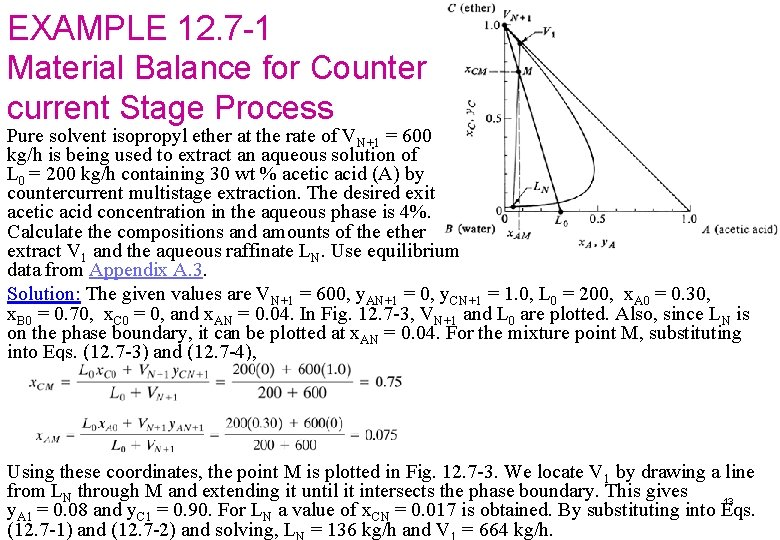EXAMPLE 12. 7 -1 Material Balance for Counter current Stage Process Pure solvent isopropyl ether at the rate of VN+1 = 600 kg/h is being used to extract an aqueous solution of L 0 = 200 kg/h containing 30 wt % acetic acid (A) by countercurrent multistage extraction. The desired exit acetic acid concentration in the aqueous phase is 4%. Calculate the compositions and amounts of the ether extract V 1 and the aqueous raffinate LN. Use equilibrium data from Appendix A. 3. Solution: The given values are VN+1 = 600, y. AN+1 = 0, y. CN+1 = 1. 0, L 0 = 200, x. A 0 = 0. 30, x. B 0 = 0. 70, x. C 0 = 0, and x. AN = 0. 04. In Fig. 12. 7 -3, VN+1 and L 0 are plotted. Also, since LN is on the phase boundary, it can be plotted at x. AN = 0. 04. For the mixture point M, substituting into Eqs. (12. 7 -3) and (12. 7 -4), Using these coordinates, the point M is plotted in Fig. 12. 7 -3. We locate V 1 by drawing a line from LN through M and extending it until it intersects the phase boundary. This gives 13 y. A 1 = 0. 08 and y. C 1 = 0. 90. For LN a value of x. CN = 0. 017 is obtained. By substituting into Eqs. (12. 7 -1) and (12. 7 -2) and solving, L = 136 kg/h and V = 664 kg/h.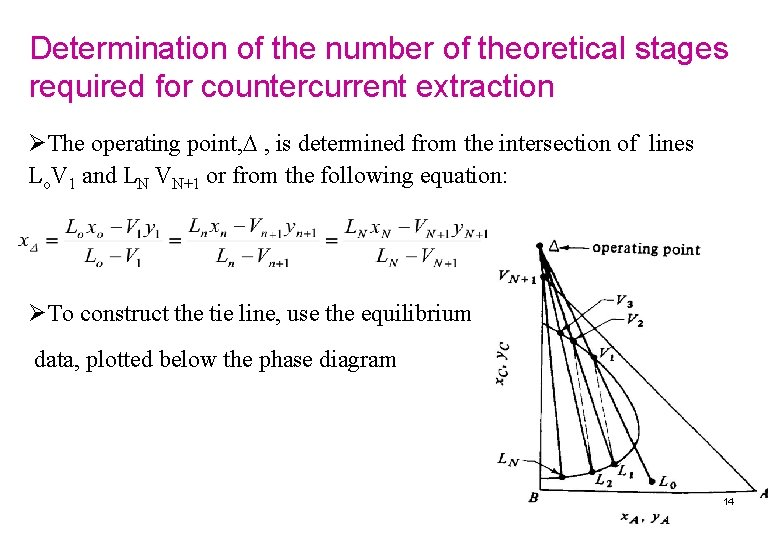Determination of the number of theoretical stages required for countercurrent extraction ØThe operating point, , is determined from the intersection of lines Lo. V 1 and LN VN+1 or from the following equation: ØTo construct the tie line, use the equilibrium data, plotted below the phase diagram 14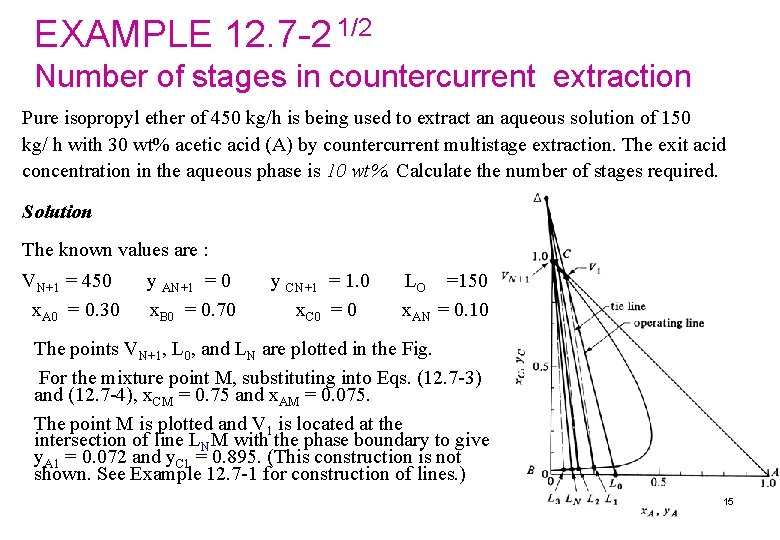EXAMPLE 12. 7 -2 1/2 Number of stages in countercurrent extraction Pure isopropyl ether of 450 kg/h is being used to extract an aqueous solution of 150 kg/ h with 30 wt% acetic acid (A) by countercurrent multistage extraction. The exit acid concentration in the aqueous phase is 10 wt%. Calculate the number of stages required. Solution The known values are : VN+1 = 450 x. A 0 = 0. 30 y AN+1 = 0 x. B 0 = 0. 70 y CN+1 = 1. 0 x. C 0 = 0 LO =150 x. AN = 0. 10 The points VN+1, L 0, and LN are plotted in the Fig. For the mixture point M, substituting into Eqs. (12. 7 -3) and (12. 7 -4), x. CM = 0. 75 and x. AM = 0. 075. The point M is plotted and V 1 is located at the intersection of line LNM with the phase boundary to give y. A 1 = 0. 072 and y. C 1 = 0. 895. (This construction is not shown. See Example 12. 7 -1 for construction of lines. ) 15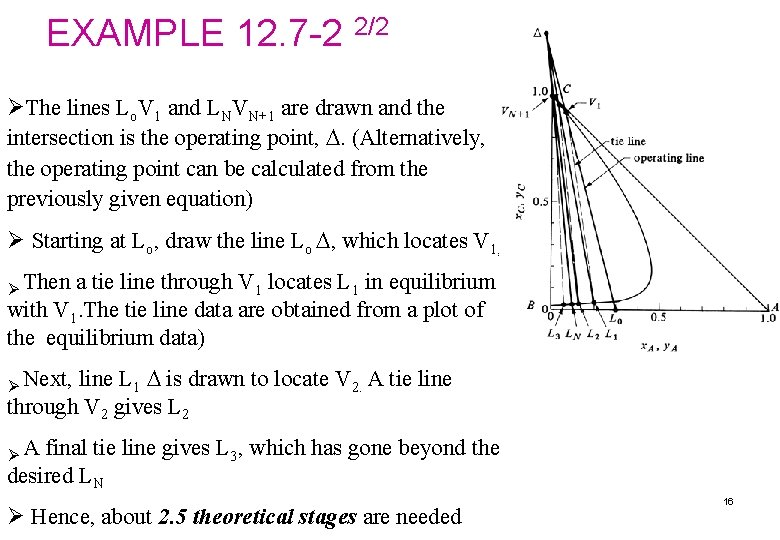EXAMPLE 12. 7 -2 2/2 ØThe lines Lo. V 1 and LNVN+1 are drawn and the intersection is the operating point, . (Alternatively, the operating point can be calculated from the previously given equation) Ø Starting at Lo, draw the line Lo , which locates V 1, . Ø Then a tie line through V 1 locates L 1 in equilibrium with V 1. The tie line data are obtained from a plot of the equilibrium data) line L 1 is drawn to locate V 2. A tie line through V 2 gives L 2 Ø Next, ØA final tie line gives L 3, which has gone beyond the desired LN Ø Hence, about 2. 5 theoretical stages are needed 16Equipment for liquid-liquid extraction 1/5 A) Mixer settlers for extraction Ø Mechanical mixer is often used to provide efficient mass transfer between two liquid phases Ø One phase is usually dispersed into the other in the form of small droplets Ø Small droplets provide large interfacial areas and faster extraction Ø The droplets must not be too small as larger settling time will be required 17Equipment for liquid-liquid extraction 2/5 B) Spray columns Ø Either the light or the heavy phase may be dispersed Ø In (a), the light phase enters from a distributor at the bottom of the column, rises through a heavier phase and finally coalesces to form liquid interface at the top of column Ø In (b) the heavier phase is dispersed, where the interface is held at the bottom of the tower 18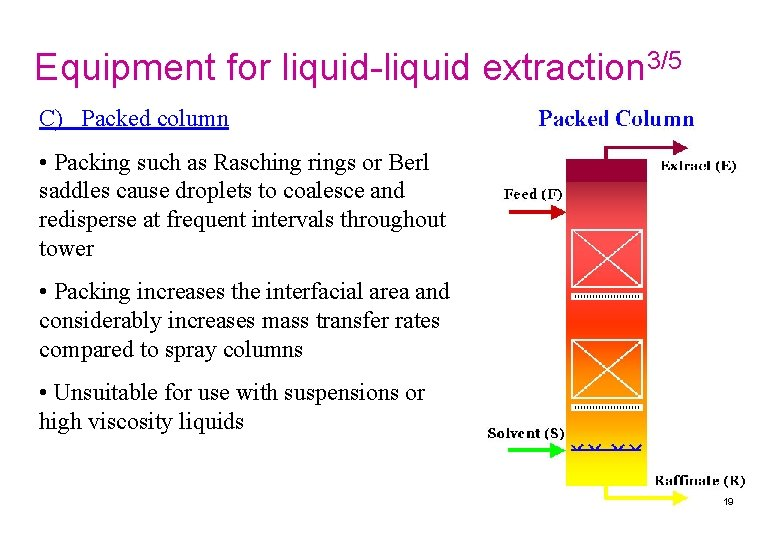Equipment for liquid-liquid extraction 3/5 C) Packed column • Packing such as Rasching rings or Berl saddles cause droplets to coalesce and redisperse at frequent intervals throughout tower • Packing increases the interfacial area and considerably increases mass transfer rates compared to spray columns • Unsuitable for use with suspensions or high viscosity liquids 19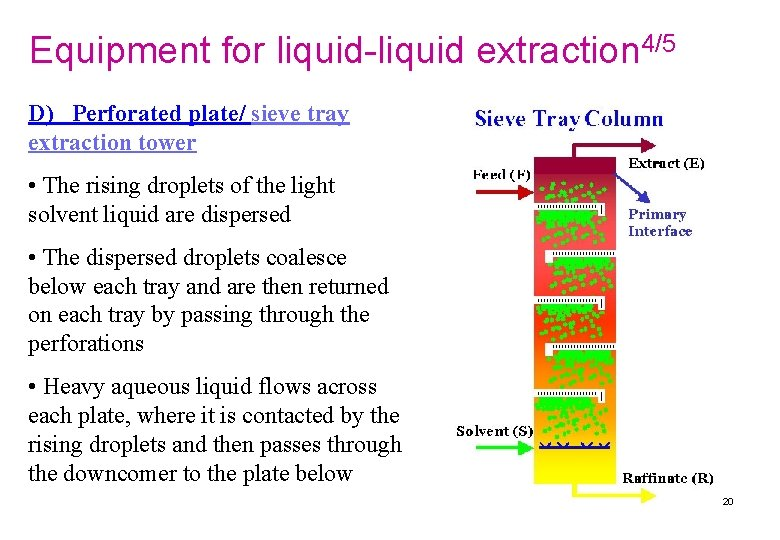Equipment for liquid-liquid extraction 4/5 D) Perforated plate/ sieve tray extraction tower • The rising droplets of the light solvent liquid are dispersed • The dispersed droplets coalesce below each tray and are then returned on each tray by passing through the perforations • Heavy aqueous liquid flows across each plate, where it is contacted by the rising droplets and then passes through the downcomer to the plate below 20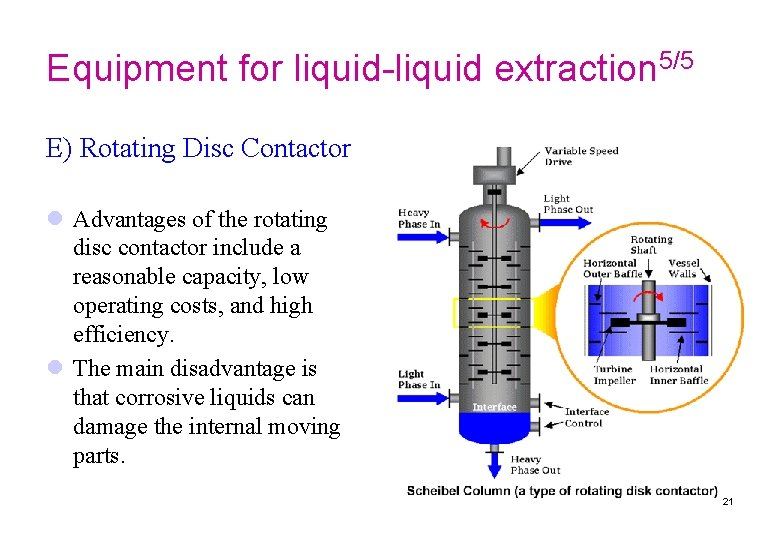Equipment for liquid-liquid extraction 5/5 E) Rotating Disc Contactor l Advantages of the rotating disc contactor include a reasonable capacity, low operating costs, and high efficiency. l The main disadvantage is that corrosive liquids can damage the internal moving parts. 21Table 12. 6 -1. Typical Performance for Several Types of Commercial Extraction Towers 22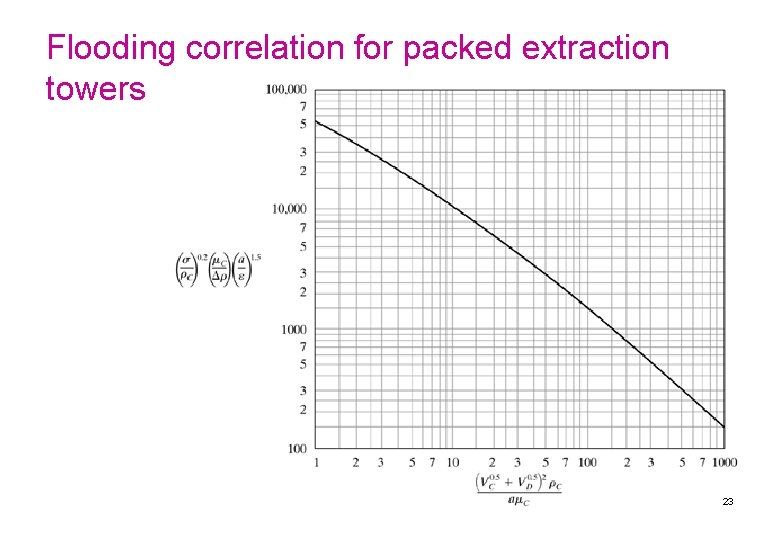Flooding correlation for packed extraction towers 23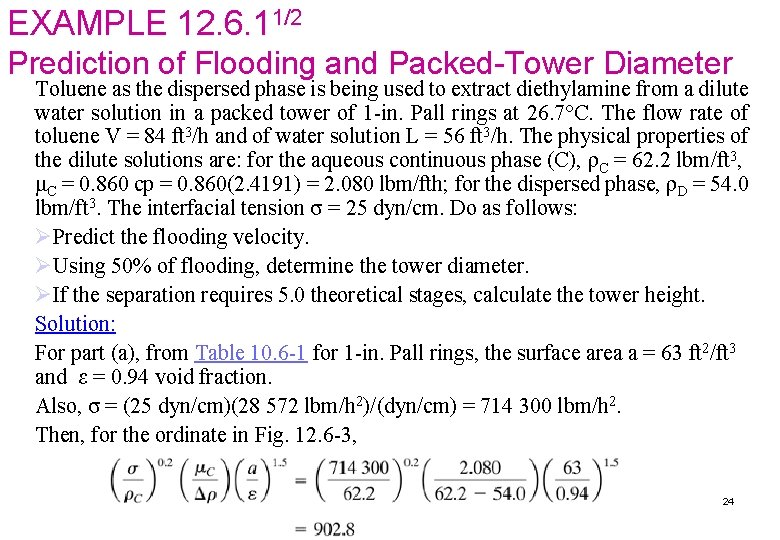EXAMPLE 12. 6. 11/2 Prediction of Flooding and Packed-Tower Diameter Toluene as the dispersed phase is being used to extract diethylamine from a dilute water solution in a packed tower of 1 -in. Pall rings at 26. 7°C. The flow rate of toluene V = 84 ft 3/h and of water solution L = 56 ft 3/h. The physical properties of the dilute solutions are: for the aqueous continuous phase (C), ρC = 62. 2 lbm/ft 3, μC = 0. 860 cp = 0. 860(2. 4191) = 2. 080 lbm/fth; for the dispersed phase, ρD = 54. 0 lbm/ft 3. The interfacial tension σ = 25 dyn/cm. Do as follows: ØPredict the flooding velocity. ØUsing 50% of flooding, determine the tower diameter. ØIf the separation requires 5. 0 theoretical stages, calculate the tower height. Solution: For part (a), from Table 10. 6 -1 for 1 -in. Pall rings, the surface area a = 63 ft 2/ft 3 and ε = 0. 94 void fraction. Also, σ = (25 dyn/cm)(28 572 lbm/h 2)/(dyn/cm) = 714 300 lbm/h 2. Then, for the ordinate in Fig. 12. 6 -3, 24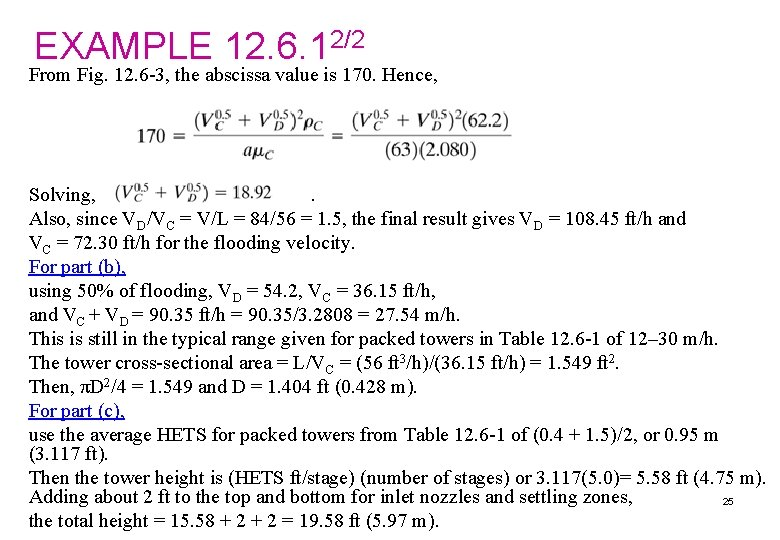EXAMPLE 12. 6. 12/2 From Fig. 12. 6 -3, the abscissa value is 170. Hence, Solving, . Also, since VD/VC = V/L = 84/56 = 1. 5, the final result gives VD = 108. 45 ft/h and VC = 72. 30 ft/h for the flooding velocity. For part (b), using 50% of flooding, VD = 54. 2, VC = 36. 15 ft/h, and VC + VD = 90. 35 ft/h = 90. 35/3. 2808 = 27. 54 m/h. This is still in the typical range given for packed towers in Table 12. 6 -1 of 12– 30 m/h. The tower cross-sectional area = L/VC = (56 ft 3/h)/(36. 15 ft/h) = 1. 549 ft 2. Then, πD 2/4 = 1. 549 and D = 1. 404 ft (0. 428 m). For part (c), use the average HETS for packed towers from Table 12. 6 -1 of (0. 4 + 1. 5)/2, or 0. 95 m (3. 117 ft). Then the tower height is (HETS ft/stage) (number of stages) or 3. 117(5. 0)= 5. 58 ft (4. 75 m). Adding about 2 ft to the top and bottom for inlet nozzles and settling zones, 25 the total height = 15. 58 + 2 = 19. 58 ft (5. 97 m).12. 7 C. Countercurrent-Stage Extraction with Immiscible Liquids If the solvent stream VN+1 contains components A and C and the feed stream L 0 contains A and B, and if components B and C are relatively immiscible in each other, the stage calculations may be made more easily. where L'= kg inert B/h, V' = kg inert C/h, y = mass fraction A in V stream, and x = mass fraction A in L stream. This Eq. (12. 7 -13) is an operating-line equation whose slope L'/V'. If y and x are quite dilute, the line will be straight when plotted on an x-y diagram. 26EXAMPLE 12. 7 -3. 1/2 Extraction of Nicotine with Immiscible Liquids. An inlet water solution of 100 kg/h containing 0. 010 wt fraction nicotine (A) in water is stripped with a kerosene stream of 200 kg/h containing 0. 0005 wt fraction nicotine in a countercurrent-stage tower. The water and kerosene are essentially immiscible in each other. It is desired to reduce the concentration of the exit water to 0. 0010 wt fraction nicotine. Determine theoretical number of stages needed. Solution: The given values are L 0 = 100 kg/h, x 0 = 0. 010, VN+1 = 200 kg/h, y. N+1 = 0. 0005, x. N = 0. 0010. The inert streams are Making an overall balance on A using Eq. (12. 7 -12) and solving, y 1 = 0. 00498. These points on the operating line are plotted in Fig. 12. 7 -6. 27EXAMPLE 12. 7 -3. 2/2 Since the operating-line equation is for dilute solutions, a straight line is drawn. The equilibrium data are plotted and the line is slightly curved. The number of stages are stepped off, giving N = 4. 5 theoretical stages. 28